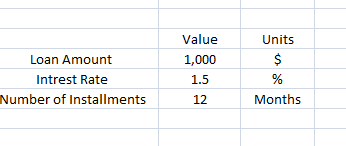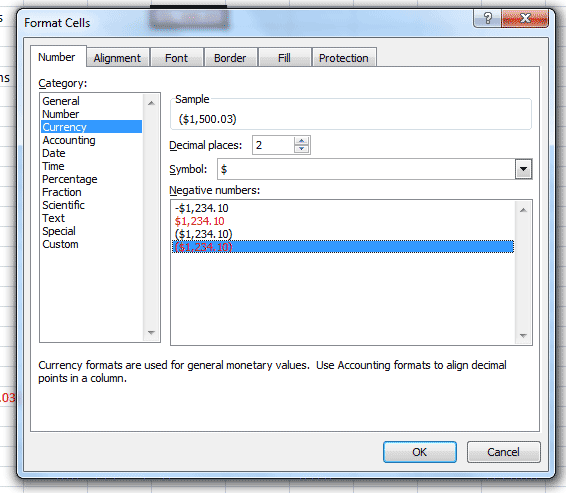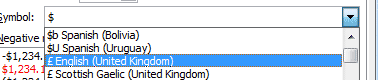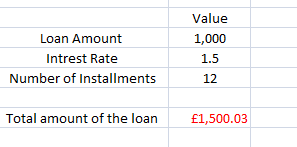# Total Amount of a Loan Using Excel

Microsoft Excel can be easily used to calculate the total amount of a loan to be repaid. The total amount to be repaid in a loan is a combination of the initial amount borrowed and the total amount of interest to be added, excel can very easily calculate the total amount to be paid using the PMT function. For the PMT function to calculate the entire loan to be repaid three bits of information are required:

rate - The interest rate of the loan expressed as a decimal.

nper - The number of installments.

pv - The amount of the loan.

The calculated value displays the total amount of the loan, not the individual monthly repayments. Always ensure that the rate and nper values are based upon the same units of time eg months, weeks or years.

## Worked Excel Example to Calculate total Loan Amount

Before using the =PMT equation set up an excel work book with the required information - interest rate, amount to be borrowed and number of installments. In the worked example below a loan of 1,000 is required which be paid back over 12 monthly installments at 1.2% monthly interest. For the excel pmt calculation the units are not required and have been added into a separate row for information.The next stage is to entre the PMS function in the formula bar. The function is:The three values to be entered are the "rate" (interest rate), "nper" number of installments and "pv" initial amount of the loan. When entering the formula always check for "," between values and ensure the cell references are surrounded by "(...)"

The function returns a value in the cell:The automatic features of the function assume that the loan will be in dollars and highlights the value in red and places it in brackets. To alter any of these features right click over the cell and click on Format CellsIn the box which opens, the format cell box, the currency and reporting method can be altered.For a different currency select the appropriate one from the list:At the reporting style altered:Click OK to altered the style of the PMT loan calculation.Related Pages